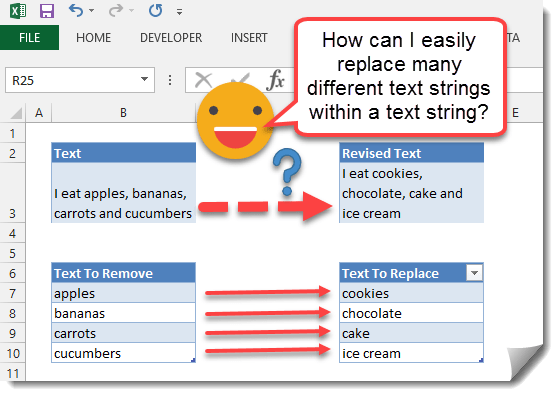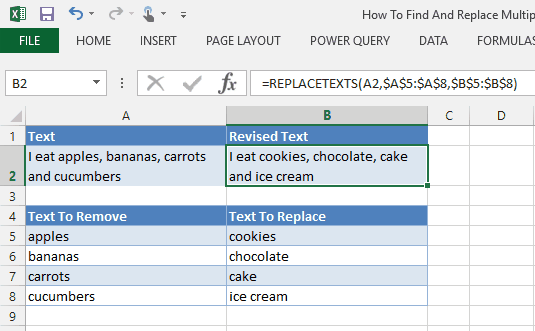In this post we’re going to explore how to find and replace multiple text strings from within another string.Excel has a great built in function called SUBSTITUTE which allows you to find one bit of text within another text string and substitute it for another bit of text.

Copy and paste this table into cell A1 in Excel

Text Revised Text
I eat apples and bananas
`=SUBSTITUTE(A2,"apples","cookies")`

In the above example we can use the SUBSTITUTE function to replace all instances of apples with cookies using the following formula.

``=SUBSTITUTE(A2,"apples","cookies")``

Copy and paste this table into cell A1 in Excel

Text Revised Text
I eat apples and bananas
`=SUBSTITUTE(SUBSTITUTE(A2,"apples","cookies"),"bananas","chocolate")`

Now if we also want to replace bananas with chocolate we could do this by using a nested SUBSTITUTE formula.

``=SUBSTITUTE(SUBSTITUTE(A2,"apples","cookies"),"bananas","chocolate")``

As we add more and more items we want to replace we need to nest more and more SUBSTITUTE functions and this will become more unmanageable. So instead we will create a user defined function in VBA to simplify this. If you want to know how to use this VBA code then read this post about How To Use The VBA Code You Find Online.

``````
Function REPLACETEXTS(strInput As String, rngFind As Range, rngReplace As Range) As String

Dim strTemp As String
Dim strFind As String
Dim strReplace As String

Dim cellFind As Range

Dim lngColFind As Long
Dim lngRowFind As Long
Dim lngRowReplace As Long
Dim lngColReplace As Long

lngColFind = rngFind.Columns.Count
lngRowFind = rngFind.Rows.Count
lngColReplace = rngFind.Columns.Count
lngRowReplace = rngFind.Rows.Count

strTemp = strInput

If Not ((lngColFind = lngColReplace) And (lngRowFind = lngRowReplace)) Then
REPLACETEXTS = CVErr(xlErrNA)
Exit Function
End If

For Each cellFind In rngFind

strFind = cellFind.Value
strReplace = rngReplace(cellFind.Row - rngFind.Row + 1, cellFind.Column - rngFind.Column + 1).Value
strTemp = Replace(strTemp, strFind, strReplace)

Next cellFind

REPLACETEXTS = strTemp

End Function
``````

This user defined function takes a text element and two ranges as input.

• strInput – this is the text you want to replace bits of text from.
• rngFind – this is a range that contains text strings you want to find in strInput.
• rngReplace – this is a range that contains text strings you want to replace items from rngFind with. The dimensions of rngFind and rngReplace must be equal or the function will return an error.

Copy and paste this table into cell A1 in Excel

Text Revised Text
I eat apples, bananas, carrots and cucumbers
`=REPLACETEXTS(A2,\$A\$6:\$A\$9,\$B\$6:\$B\$9)`

With this user defined function we can easily take care of replacing multiple texts using a simple looking formula without nesting multiple SUBSTITUTE functions.

``=REPLACETEXTS(A2,\$A\$6:\$A\$9,\$B\$6:\$B\$9)``

Where \$A\$6:\$A\$9 is a range containing the text we want to remove (apples, bananas, carrots and cucumbers) and \$B\$6:\$B\$9 is a range containing the text we want to replace them with (cookies, chocolate, cake, ice cream).